# solid geometry

2 posts / 0 new
Renz Kolin Gaba...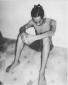solid geometry

Imagine a cube to measure 5 units on an edge, and to have its total surface area blue. Without the aid of a figure or a cubical object answer the following questions.
(A) how many times must you cut completely through the cube to make cubes with measure 1 unit on an edge?
(B) How many of the cubes of question (A) will have:
(a) Three blue faces?
(b) Two blue faces?
(c) One blue face?
(d) No blue faces?

fitzmerl duronI like figures....heheheh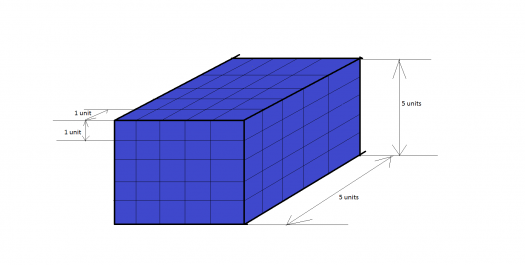$\mathbf{For \space A.)}$

You need to cut through the cube $\color{blue}{12 \space times}$ to get $1 \times 1 \space$ cubes.

$\mathbf{For \space B.)}$

$\mathbf{a.)}$

To get how many $3$ blue faced cubes, look at the figure below.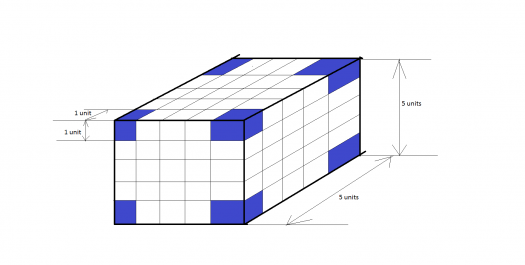It was found out that there are $\color{blue}{4+4 = 8 \space 1\times 1 \space cubes}$ having 3 blue faces.

$\mathbf{b.)}$

To get how many $2$ blue faced cubes, look at the figure below.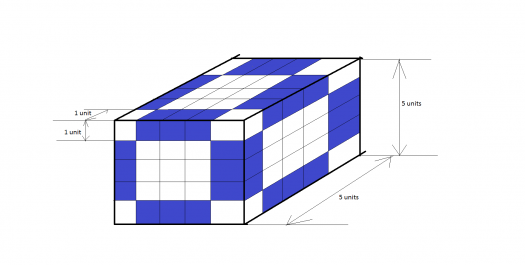It was found out that there are $\color{blue}{(12 \times 2) + (6 \times 2) = 36 \space 1\times 1 \space cubes}$ having 2 blue faces.

$\mathbf{c.)}$

To get how many $1$ blue faced cubes, look at the figure below.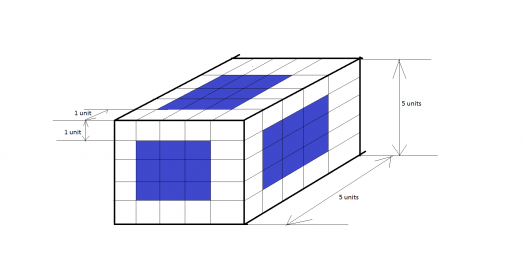It was found out that there are $\color{blue}{9 \times 6 = 54 \space 1\times 1 \space cubes}$ having 1 blue face.

$\mathbf{d.)}$

To get how many $0$ blue faced cubes, look at the figure below.It was found out that there are $\color{blue}{125 - (8 + 36 + 54) = 27 \space 1\times 1 \space cubes}$ having no blue face. There are $125 \space 1 \times 1 \space$ cubes in the $5 \times 5 \space$ cube being cut into $1 \times 1 \space$ cubes. There are $8$ 3-blue faced cubes, $36$ 2-blue faced cubes and $54$ 1-blue faced cubes. The no-blue colored $1 \times 1 \space$ cubes were hidden inside the $5 \times 5 \space$ cube.

Alternate solutions are encouraged...

Subscribe to MATHalino on